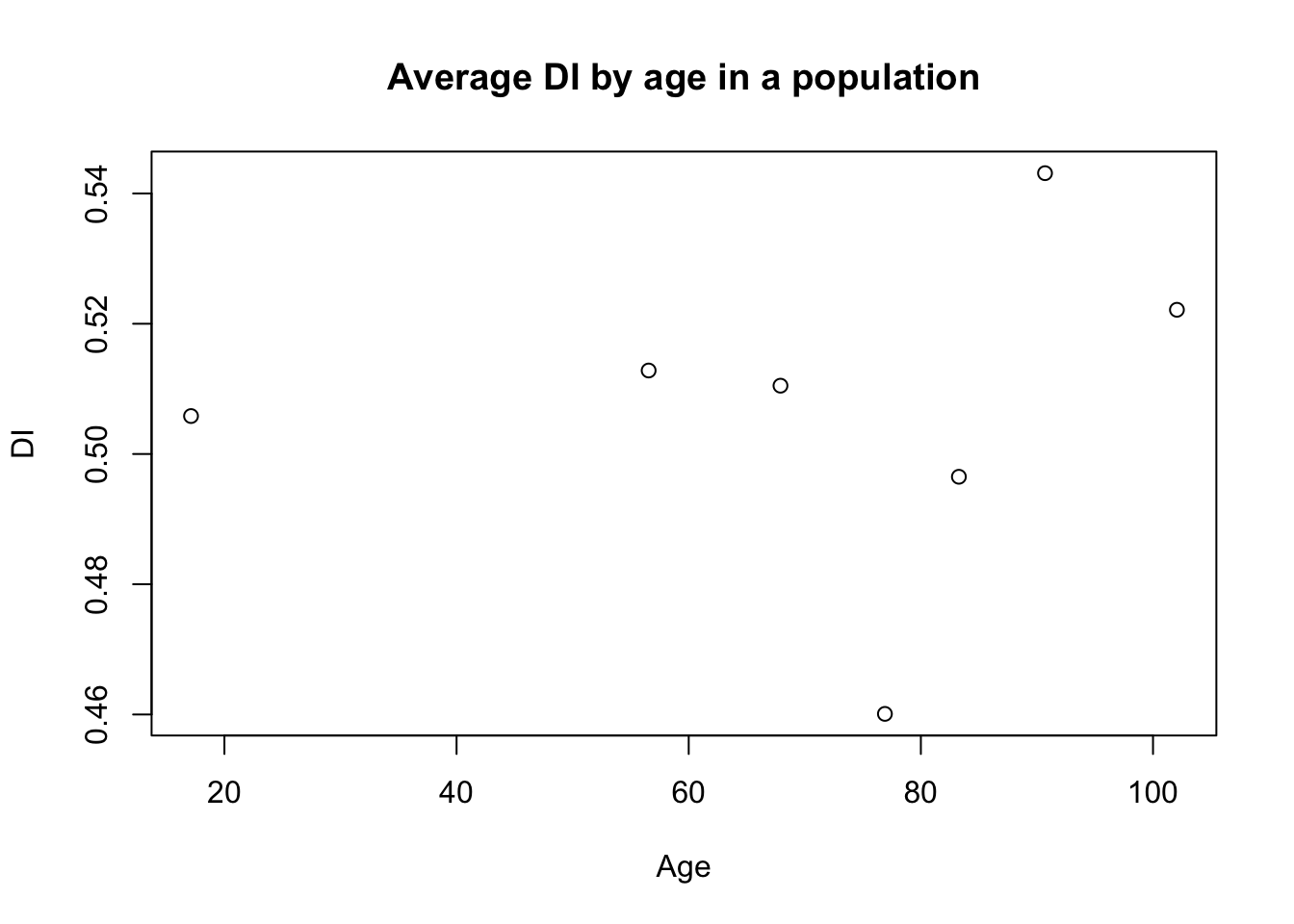Overview

Deficit (frailty) Index (DI) summarizes a range of deficits from in surveys or clinical data sets. “Recalling that frailty is an age-associated, nonspecific vulnerability, we consider symptoms, signs, diseases, and disabilities as deficits, which are combined in a frailty index. An individual’s frailty index score reflects the proportion of potential deficits present in that person, and indicates the likelihood that frailty is present. Although based on a simple count, the frailty index shows several interesting properties, including a characteristic rate of accumulation, a submaximal limit, and characteristic changes with age in its distribution. The frailty index, as a state variable, is able to quantitatively summarize vulnerability. Future studies include the application of network analyses and stochastic analytical techniques to the evaluation of the frailty index and the description of other state variables in relation to frailty.” 

1. Frailty in Relation to the Accumulation of Deficits. Available from: https://www.researchgate.net/publication/6204727_Frailty_in_Relation_to_the_Accumulation_of_Deficits [accessed Jun 11, 2017].

Examples

Basic usage

library(di)
# Simulate database of N individuals:
N <- 1000
dd <- data.frame(subj=seq(1:N),
var1=rbinom(N,1,.5),
var2=rbinom(N,1,.5),
var3=rbinom(N,1,.5),
age=rnorm(N, 80, 20))
# Calculate DI
ddi <- di(dd, c("var1", "var2", "var3"))

Rescaling

By default, values are rescaled to the interval [0,1]. If you do not want this, you can set the rescale flag to FALSE:

ddi <- di(dd, c("var1", "var2", "var3"), rescale = FALSE)

Custom rescaling

Sometimes it needs to apply a custom scaling. For example if values 0 and 1 incorrectly represent the reality, the user can apply his own scale: 0 –> 0.2 and 1 –> 0.8. To do this, he needs to use a special grammar: . Example below shows this situation:

ddi <- di(dd, c("var1", "var2", "var3"), rescale.custom = c("var1:0.2:0.8", "var3:0.3:0.7"))

Avoiding rescaling for some columns

Please use rescale.avoid parameter:

ddi <- di(dd, c("var1", "var2", "var3"), rescale.avoid = c("var2"))

Inverting

The option invert allows inverting values of some columns (user-defined). invert is NULL by default.

ddi <- di(dd, c("var1", "var2", "var3"), rescale = FALSE, invert=c("var1", "var3"))

Plotting

ddi <- di(dd, c("var1", "var2", "var3"), age="age", visible=TRUE)